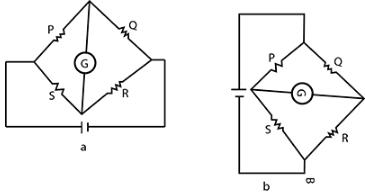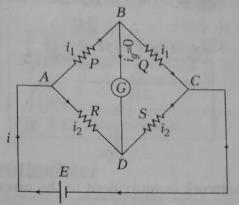Courses

# Test: Electrical Appliances

## 10 Questions MCQ Test Physics Class 12 | Test: Electrical Appliances

Description
This mock test of Test: Electrical Appliances for JEE helps you for every JEE entrance exam. This contains 10 Multiple Choice Questions for JEE Test: Electrical Appliances (mcq) to study with solutions a complete question bank. The solved questions answers in this Test: Electrical Appliances quiz give you a good mix of easy questions and tough questions. JEE students definitely take this Test: Electrical Appliances exercise for a better result in the exam. You can find other Test: Electrical Appliances extra questions, long questions & short questions for JEE on EduRev as well by searching above.
QUESTION: 1

### Sensitivity of potentiometer can be increased by

Solution:

Sensitivity of the potentiometer means the smallest potential difference it can measure. It can be increased by reducing the potential gradient. The same is possible by increasing the length of the potentiometer. Hence the correct option is option C.

QUESTION: 2

### In a potentiometer a cell of e.m.f 2V gives a balance point at 30cm. If the cell is replaced by another cell and the balance point shifts to 60cm.What is the e.m.f of the other cell?​

Solution:

Changes(if required):
Solution:
We can use the formula E1/E2=L1/L2
E1=2v,E2=?,l1=30,l2=60
2/E2=30/60
E2=120/30=4v

QUESTION: 3

### When the position of cell and galvanometer in a Wheatstone bridge is inter-changed, its balanced condition

Solution:

For balanced Wheatstone bridge which is shown in figure a, P/Q​=S/R​
If we interchange the cell and galvanometer then circuit becomes as shown in figure b.
and balanced condition, P/S​=Q​/R⇒P/Q​=S/R​
Thus, balanced point remains unchanged.QUESTION: 4

An electric bulb is rated 220 V and 100 W. Power consumed by it operated on 110 V is

Solution:

Power, P= V2/R​
R= V2/P​
R=220×220/100 ​=484Ω
The current flow in the circuit,
i= V′/R​ (V′= supplied voltage)
i=110/484​=0.2272A
The voltage drop across the bulb is 110V
The power consumed by the bulb,
Pb​=V2/R​=110×110​/484
Pb​=25W
The correct option is C.

QUESTION: 5

Meter Bridge or Slide Wire Bridge is a practical form of

Solution:

The meter bridge principle is based on the Wheatstone bridge circuit which says that if at any point of(of a wire), the ratio of two resistances (say R1) is equal to the ratio of another two resistance (say R3 and R4 where R4is the unknown resistance),Then there shall be no flow of current at that point between those points and the edge containing the resistances (R1/R2 and R3/R4) therefore, applying it to the meter bridge, at such point, the galvanometer will show zero defection.

QUESTION: 6

When Wheatstone bridge is in balance condition, the current through galvanometer will be

Solution:The bridge is said to be balanced when deflection in galvanometer is zero(Ig=0), i.e., no current flows through the galvanometer(branch BD).In the balanced condition,
P/Q=R/S
On mutually changing the position of the cell and galvanometer, this condition will not change.

QUESTION: 7

The resistance in ohms in the four arms of a Wheatstone bridge are as follows. In which combination of the following is the bridge balanced?​

Solution:

Wheat stone bridge follows

P/Q = R/S

So 2/3 = 4/6

QUESTION: 8

Two bulbs A and B are rated 100 W, 120 V and 10 W, 120 V respectively. They are connected across a 120 V source in series. Calculate the current through each bulb.​

Solution:

Bulb A,
PA=100w
VA=120v
We know that, P=VI
So, I=P/V=100w/120v=5/6A=0.83A
Bulb B,
PB=100w
VB=120v
We know that, P=VI
So, I=P/V=10w/120v=1/12A=0.083A
Bulb A will consume more energy than bulb B when they are connected in parallel.

QUESTION: 9

Wheatstone bridge is used to measure

Solution:

When the variable resistor is adjusted, then the current in the galvanometer becomes zero, the ratio of two two unknown resistors is equal to the ratio of value of unknown resistance and adjusted value of variable resistance. By using a Wheatstone Bridge the unknown electrical resistance value can easily measure.

QUESTION: 10

The Wheatstone bridge and its balance condition provides a practical method for the determination of

Solution:

A wheatstone bridge is an electrical bridge consisting of two branches of a parallel circuit joined by a galvanometer and used for determining the value of an unknown resistance in one of the branches.